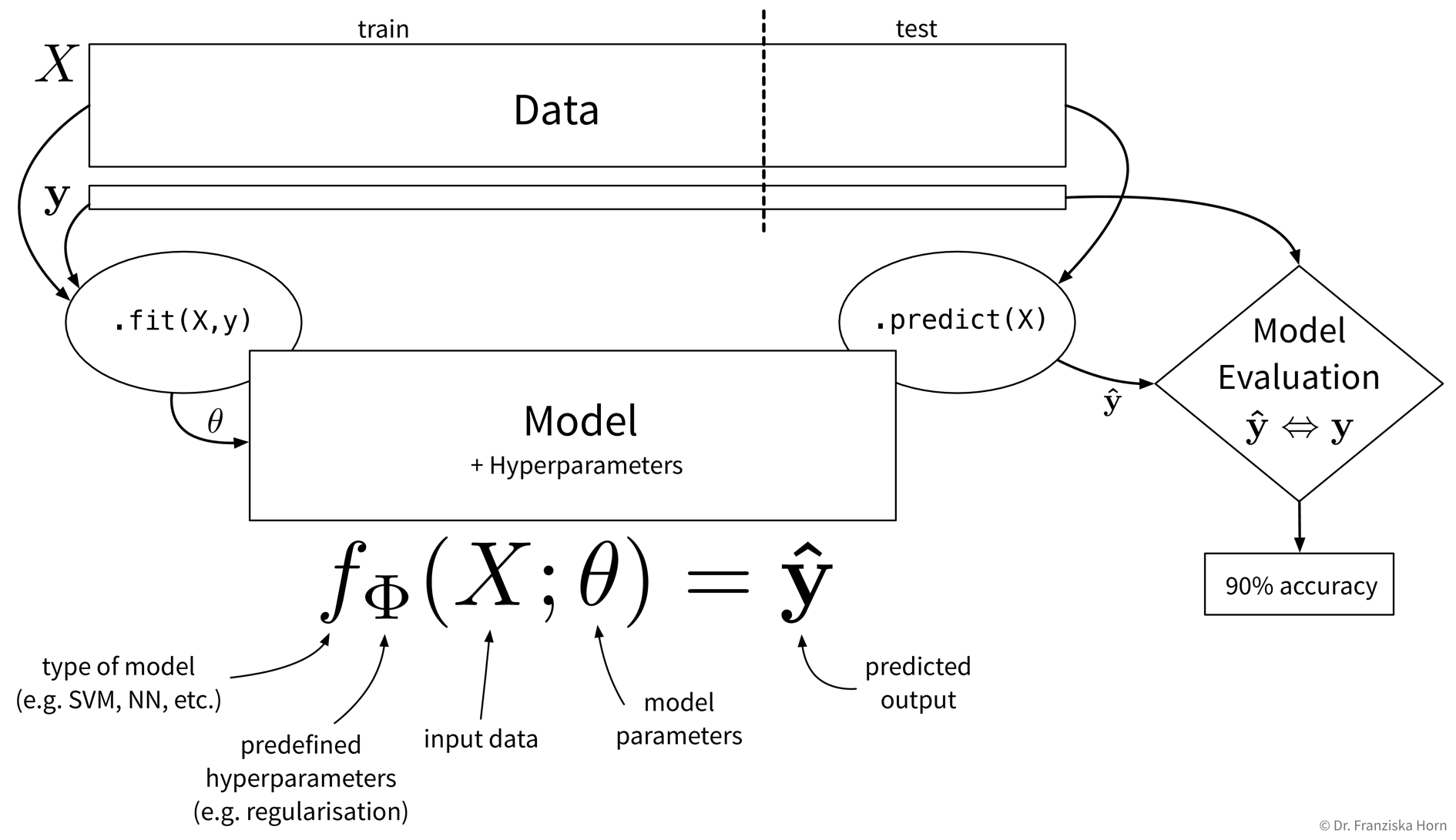## Supervised Learning Basics

Now that we’ve surveyed the different unsupervised learning algorithms, let’s move on to supervised learning:Supervised learning in a nutshell (with scikit-learn):First, the available data needs to be split into a training and test part, where we’re assigning the majority of the data points to the training set and the rest to the test set. (Please note that, as an exception, in this graphic, $X$ and $\mathbf{y}$ are rotated by 90 degrees, i.e., the features are in the rows and the data points are in the columns.) Next, we need to decide on the type of model that we want to use to describe the relationship between the inputs $X$ and the outputs $\mathbf{y}$ and this model also comes with some hyperparameters that we need to set (which are passed as arguments when instantiating the respective sklearn class). Then we can call the `.fit(X_train, y_train)` method on the model to learn the internal model parameters by minimizing some model-specific objective function on the training data. Now the model is ready to generate predictions for new data points, i.e., by calling `.predict(X_test)`, we obtain the predicted values $\mathbf{\hat{y}}$ for the test points. Finally, to get an estimate of how useful the model will be in practice, we evaluate it by comparing the predicted target values of the test set to the corresponding true labels.

In the following sections, we introduce the different approaches to supervised learning and explain when to use which kind of model, then discuss how to evaluate and select the hyperparameters of a supervised learning model.